# KSEEB Solutions for Class 8 Maths Chapter 14 Introduction of Graphs Ex 14.2

In this chapter, we provide KSEEB SSLC Class 8 Maths Chapter 14 Introduction of Graphs Ex 14.2 for English medium students, Which will very helpful for every student in their exams. Students can download the latest KSEEB SSLC Class 8 Maths Chapter 14 Introduction of Graphs Ex 14.2 pdf, free KSEEB SSLC Class 8 Maths Chapter 14 Introduction of Graphs Ex 14.2 pdf download. Now you will get step by step solution to each question.

### Karnataka State Syllabus Class 8 Maths Chapter 14 Introduction of Graphs Ex 14.2

Question 1.
Draw the graphs of the following straight lines.
(i) y = 3 – x
(ii) y = x – 3
(iii) y = 3x – 2
(iv) y = 5 – 3x
(v) 4y = – x + 3
(vi) 3y = 4x + 1
(vii) x = 4
(vii) 3y = 1
(i) y = 3 – x

When x = 0,                           y = 3 – 0 = (3)
When x = 1,                           y = 3 – 1 = (2)
Whenx = 2,                             y = 3 – 2 = (1)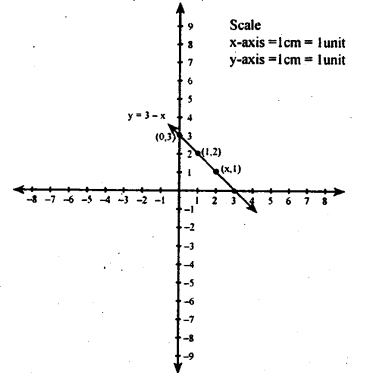(ii) y = x – 3

When x = 0,                           y = 0 – 3 = (- 3)
When x = 1,                        y = 1 – 3 = (- 2)
Whenx = 2,                            y = 2 – 3 = (- 1)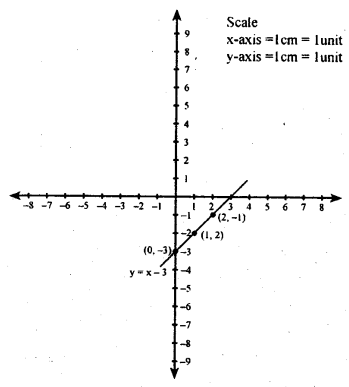(iii) y = 3x – 2

When x = 0,                           y = 3(0) – 2 = (- 2)
When x = 1,                        y = 3(1) – 2 = (1)
Whenx = 2,                            y =3(2) – 2 = (4)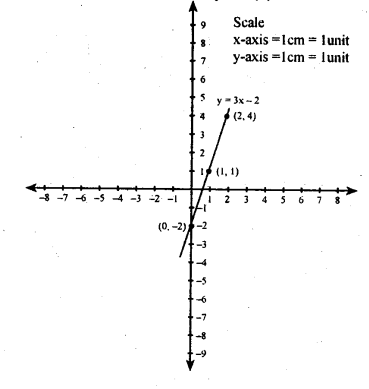(iv) y = 5 – 3x

When x = 0,                y = 5 – 3(0) = 5
When x = 1,                y = 5 – 3(1) = 2
Whenx = 2,                y = 5 – 3(2) = 5 – 6 = -1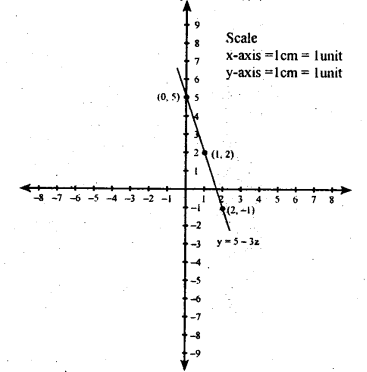(v) 4y = – x + 3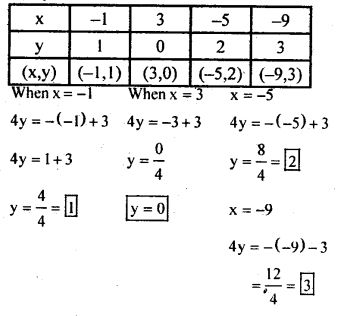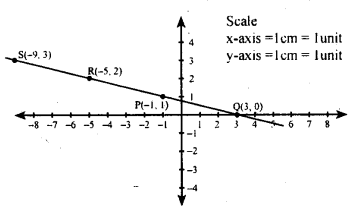(vi) 3y = 4x + 1

(vii) x = 4

(vii) 3y = 1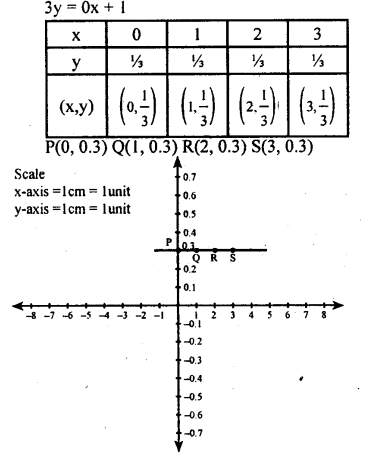Question 2.
Draw the graph of yx=y+1x+2
When x = 1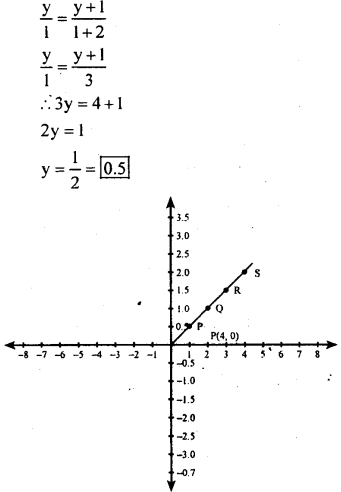Question 3.
Determine the equation of the line in each of the following graphs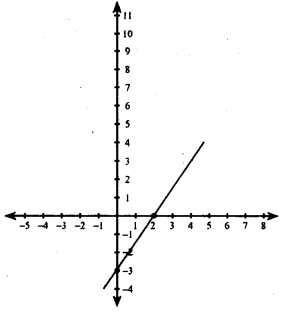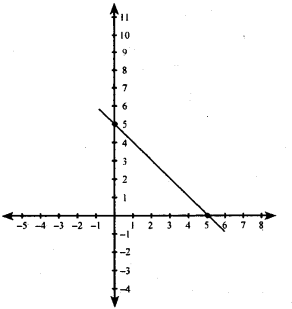(i) Let the equation be y = ax + b
We find that the lines passes through (2,0)
i. e., when x = 2, y = 0
∴ y = ax+b
0 = ax + b
0 = a(2) + b
0 = 2a + b
∴ b = – 2a
The line passes through (4,3) also
i. e., when x=4 , y = 3
∴ y = ax + b
3 = a(4) + b
3 = 4a + b
3 = 4a + (-2a)
3 = 4a = 2a
a = 32 b = – 2a = −2⋅32 = -3
y = ax + b y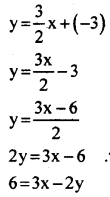The equation is 3x – 2y = 6

(ii) Let the equation be y = ax + b
we find that the line passes through (5,0) i.e., when x = 5, y = 0
∴ y = ax + b
0 = a(5) + b
0 = 5a + b
b = -5a
The line passes through (0 , 5)also
i.e.,when x = 0, y = 5
y = ax + b
5 = a(0) + b
5 = 0 + b
5 = 0 + (-5a)
5 = -5a
∴ a =$\frac { -5 }{ 5 }$
a = -1
b = -5a = -5(-1) = 5 b = 5
b = 5
y=ax + b
y = – 1 x + 5 y = – x + 5 y + x = 5
The equation of the line of is y + x = 6

Question 4.
A boat is moving in a river, downstream, whose stream has speed 8km/ hr. the speed of the motor of the boat is 22km/hr. Draw the graph of the distance covered by the boat versus an hour.
Speed of the boat downstream = 22 + 8 = 30 km/hr
y = 30x

Question 5.
Find the point of intersection of the straight lines 3y + 4x = 7 and 4y + 3x = 7, by drawing their graphs and looking for the point where they meet.
3y + 4x = 7
3y = 7 -4x
y−7−4×3

When x=1, y−7−4×3 = 7−43=33=1
When x = 4,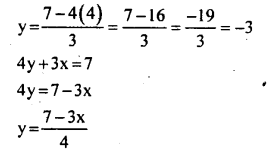All Chapter KSEEB Solutions For Class 8 maths

—————————————————————————–

All Subject KSEEB Solutions For Class 8

*************************************************

I think you got complete solutions for this chapter. If You have any queries regarding this chapter, please comment on the below section our subject teacher will answer you. We tried our best to give complete solutions so you got good marks in your exam.

If these solutions have helped you, you can also share kseebsolutionsfor.com to your friends.

Best of Luck!!